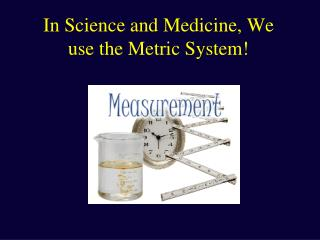# In Science and Medicine, We use the Metric System! - PowerPoint PPT PresentationDownload PresentationIn Science and Medicine, We use the Metric System!

In Science and Medicine, We use the Metric System!
Download Presentation## In Science and Medicine, We use the Metric System!

- - - - - - - - - - - - - - - - - - - - - - - - - - - E N D - - - - - - - - - - - - - - - - - - - - - - - - - - -
##### Presentation Transcript

1. In Science and Medicine, We use the Metric System!

2. Dimensions • Unit of length: meter • Unit of mass: gram • Unit of volume: liter

3. SI Base Units of Measurement • Mass = the gram; g • 1 gram ≈ 1/28 ounce • Length = the meter; m • 1 meter ≈ 1.094 yard • Volume = the liter; l • defined as 1000 cubic centimeters • 1 liter ≈ 1.057 quart • Temperature = degrees Celsius; oC

4. Prefixes • The metric system uses prefixes to represent units • smaller and larger than the fundamental unit by factors of 10. • For example, a centimeter is 1/100 of a meter, and a kilometer is 1000 times a meter • For very large numbers, we use the powers-of-ten notation, e.g. 102 = 100, 106 = 1,000,000, 10-2 = 0.01

5. Prefixes • One of the clever ideas behind the system was to use only multiples of ten.

6. Converting Between Metric Units • To convert from a LARGER unit of measure to a SMALLER unit of measure, MULTIPLY by the “order-of-magnitude difference” between the units. • Example: 1.2 mg = ______ g • 1 mg = 1000 g • 1.2 mg = 1.2 x 1000 g = 1200 g • Remember to check if your answer is logical. • It should take a GREATER NUMBER of SMALL UNITS to equate to a LESSER NUMBER of LARGE UNITS. • A microgram is smaller than a milligram (by a factor of 1000), so a given number of milligrams should be equal to many more micrograms (1000 times as many).

7. Converting Between Metric Units • To convert from a SMALLER unit of measure to a LARGER unit of measure, DIVIDE by the “order-of-magnitude difference” between the units. • Example: 250 ml = ______ l • 1 l = 1000 ml • 250 ml = 250 ÷ 1000 l = 0.25 l • Is your answer is logical? • It should take a LESSER NUMBER of LARGE UNITS to equate to a GREATER NUMBER of SMALL UNITS. • A liter is larger than a milliliter (by a factor of 1000), so a given number of milliliters should be equal to fewer liters (1/1000 as many).

8. 1 kilometer = ~0.62 or ~5/8 mi 1 meter = 1.09 yard = 39.4 in 1 centimeter = ~4/10 inch 1 angstrom = 1/10 nm or 10-10m 1 mile = ~1.6 km 1 yard = ~0.91 m 1 foot ~30.5 cm 1 inch = ~2.54 cm Thinking in Metric Units of Length

9. 1 liter = ~1.06 quart 1 milliliter = 1 cubic cm (1cc) ~"a thimble full" Examples 1 teaspoon ≈ 5ml 12 oz. soda ≈ 360 ml 2-liter bottle of soda 1 gallon = ~3.8 l 1 quart = ~0.95 l 1 pint = ~0.47 l 1 cup = ~240 ml 1 fluid oz. ~30 ml Thinking in Metric Units of Volume 2 liters~1/2 gal.

10. 1 kilogram = 2.2 pounds 1 gram = ~"a thimble full" of water Examples 70 kg human = 154 lbs. 1 ml of water weighs ~1 g 1 pound = 454 g 1 ounce = ~28 g Thinking in Metric Units of Mass

11. Estimate • The length of: • your bare foot • your driveway • The volume of: • a coffee cup • a bottle of shampoo • The mass of: • a steak • a dog

12. Celsius: The Metric Temperature Scale 100C 212F • Also called the centigrade scale. • Definitions • 0 Celsius = 32 Fahrenheit = water freezes • 100 Celsius = 212 Fahrenheit = water boils • Therefore, 1C = 1.8F • Conversions •  Celsius = 5/9 x ( Fahrenheit - 32) •  Fahrenheit = (9/5 x  Celsius) + 32 0C 32F

13. Thinking in Celsius • -10 Celsius = frigid (14 F) • 0 Celsius = cold (32 F) • 10 Celsius = cool (50 F) • 20 Celsius = comfortably warm (68 F) • 30 Celsius = hot (86 F) • 40 Celsius = very hot (104 F) • What would you wear outside in tempsf: • 10C, 0C, 10C, 20C, 30C?